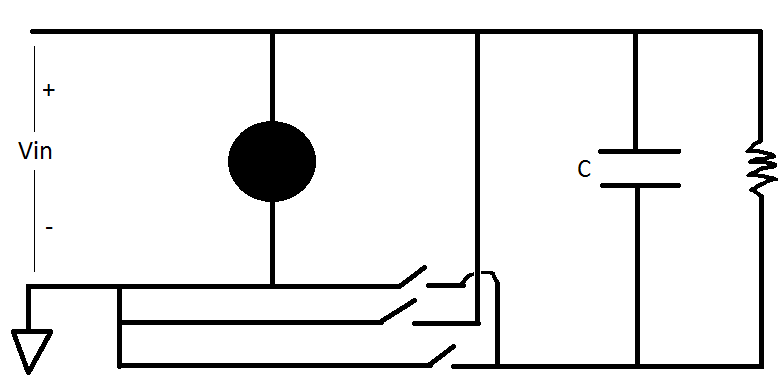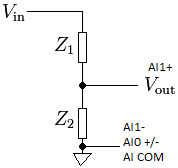# 并联的电容对电压读数有什么影响？`i = C(dV/dt)          (1)`

`V = iR          (2)`

`(V/R)/C = dV/dt          (3)`Z1 = 750 KΩ
Z2 = 75 KΩ
AI0 = 0 V
AI1 = 0.41 V
AI0 = 0 V
AI1 = 0.41 V
Z1 = 10 MΩ
Z2 = 1 MΩ
AI0 = 0 V
AI1 = 0.41 V
AI0 = 0 V
AI1 = 0.15 – 0.20 V

(4.5 V/1X106)/100X10-12 = dV/dt = 45,000 V/s = 45 mV/µs

45 mV/µs x 4 µs = 180 mV = 0.18 V

KnowledgeBase 49F73C4G: What is the Difference Between Crosstalk and Ghosting?